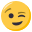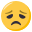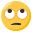# Class template specialization: How to avoid code duplication?

• Hello!

Let us consider the following example:

``````template<int N> class C
{
[...Members and methods...]
};

template<> class C<0>
{
[...The same members and methods as in generic template...]

C const &NewMethod(void){return *this;}
};
``````

Is there a possibility to avoid duplication of members and methods of the generic template in the specialized template?

• yes, there is. (it's kind of a workaround, though)

use inheritance. for example: define a common base class with all the methods you need in every derived class. or build a class hierarchy which accomplishes exactly that.

(or, don't use specialization at all. after all, if the specialized class has the same interface as the generic one, it is difficult to speak of it as "special" - that is we don't know anything about your design. the more information about it, the more appropriate the solutions will be)

• You could put all shared members into a base class an inherit both your template and the specialization from it - so you only need to duplicate the constuctors of your class:

``````template<int N>
class C_base
{
// shared members and methods
};

template<int N>
class C : public C_base<N>
{
//here you only have to define your ctor's and redirect them to their C_base counterparts
};

template<>
class C<0> : public C_base<0>
{
//again you need your ctor's here
C const& NewMethod() {return *this;}
};
``````

• you only need to duplicate the constuctors of your class
I also need to duplicate operator= and destructor (if I introduced some new members need to be destructed in the specialized class)I think I cannot use the common base class for the following reason: I need these classes to simulate behaviour of the built-in type "double". So, most of my methods are operators which return the value of the C class. If I write them in the C_base class then... what type it should receive and return ?

Here is more concrete example:

``````template<int N> class C
{
private:
double x;
public:
inline C(void): x(0.0) {;} //Default constructor
inline C(double const x): x(x) {;} //Custom constructor

inline C operator+(C const &c) const {return C(x+c.x);}
//... Other operators written in similar way
};

template<> class C<0>
{
inline C(void): x(0.0) {;} //Default constructor
inline C(double const x): x(x) {;} //Custom constructor

inline C operator+(C const &c) const {return C(x+c.x);} //operators are DUPLICATED
//... Other operator written in similar way

inline operator double(void) const {return x;} //New method
};
``````

The problem is if I write

``````inline C operator+(C const &c) const {return C(x+c.x);}
``````

in the C_base class:

``````inline C_base operator+(C_base const &c) const {return C_base(x+c.x);}
``````

then it will return value that cannot be converted to double because the "operator double" is in the derived class C<0>.

• Are you sure you need a template for this case? Where in this class is the template parameter used?

(btw, many operator overloads can be supported as global functions, so you don't need to duplicate them for your specialzations)

• I have edited my previous post: added some additional text at the end.

I need template here to compile-time control dimensions of physical values (for example, meters):

Meters*Meters = Meters^2

So the following code should not compile:

``````typedef C<1> meters;
...
meters a;
a=a*a+a; //Physical-meaningless formula!
``````

All is work for me now, but I am tired to simultaneosly modify 2 pieces of mostly similar code.

If I will not find something better, I will use #define• First - nearly every operator can be used as a global method, so your class only needs a default ctor and an explicit ctor taking a double - and maybe some explicit value getter. operator= and copy-ctor can be generated by your compiler (your measure class doesn't have any members which need extra attantion).

Second - your base class could get some additional template parameters - just look out for "mixin".

Third - maybe you take a look at this page - building physical values based on boost:mpl.

• as CStoll said, you could switch to global operators

//edit: too late.

• I don't see why it would be necessary to use any template specialization here:

``````template <int m, int s, int kg> class si
{
public:
explicit si(double v = 0.0) :
m_value(v)
{}

si operator +(si const& other)
{ return si(m_value + other.m_value); }

si operator -(si const& other)
{ return si(m_value - other.m_value); }

template <int m2, int s2, int kg2>
si<m + m2, s + s2, kg + kg2> operator * (si<m2, s2, kg2> const& other)
{ return si<m + m2, s + s2, kg + kg2>(m_value * other.m_value); }

template <int m2, int s2, int kg2>
si<m - m2, s - s2, kg - kg2> operator / (si<m2, s2, kg2> const& other)
{ return si<m - m2, s - s2, kg - kg2>(m_value / other.m_value); }

// ...
private:
double m_value;
};

typedef si<1, 0, 0> meters;
typedef si<0, 1, 0> seconds;
typedef si<0, 0, 1> kilograms;
typedef si<2, 0, 0> square_meters;
typedef si<1, -1, 0> meters_per_second;
// ...

void foo()
{
meters a(123);
meters b(10);
square_meters m2 = a * b;
}
``````

Of course if you define an implicit ctor to allow conversion from a double, or an operator double to allow conversion to a double (or even both), then many implicit conversions will be possible even though they are "unsafe" (semantically).

The worst ones should be prohibited by the "only one user-defined conversion" rule, but if I needed a class like that I'd rather go without any implicit conversion at all.

• The specialization is necessary because si<0,0,0> shold be equal to `double` , and others should not be equal. So, si<0,0,0> should have `operator double` , constructor from double and other members in addition to members that are already in `si<a,b,c>` , where a!=0, b!=0 or c!=0.

• I will use global operators.

Thank you!

• One thing you could do is explicitly disqualify si<0,0,0> (e.g. by adding a non-compilable specialisation for si<0,0,0>) and provide the "normal" templates with methods that yeld doubles for the special cases where all parameters would become 0, e.g.:

``````template <int m, int s, int kg> class si
{
public:
explicit si(double v = 0.0);

//Fundamental operators:
si operator- () { return si(-m_value); }
si& operator+= (si const& rhs) {m_value += rhs.m_value; };
si<-m,-s,-kg> inverse() {return si<-m,-s,-kg>(1/m_value);}
//Attention: operator*= only with double (others change dimensions)
si& operator*= (double rhs) {m_value *= rhs; };

//Subtraction and division in terms of Addition and Multiplication:
si& operator-= (si const& rhs) {*this += -rhs; };
si& operator/= (double rhs) {*this *= 1/rhs; };

//Binary operators in terms of the unary ones above
const si operator+ (si const& rhs) { return si(*this) += rhs; }
const si operator- (si const& rhs) { return si(*this) -= rhs; }
const si operator* (double rhs) { return si(*this) *= rhs; }
const si operator/ (double rhs) { return si(*this) /= rhs; }

//now, here goes the multiplication:
const double operator*(si<-m,-s,-kg> const& rhs) {return m_value*rhs.m_value;}

template<int m2, int s2, int kg2>
const si<m+m2,s+s2,kg+kg2> operator*(si<m2,s2,kg2> const& rhs)
{return si<m+m2,s+s2,kg+kg2>(m_value*rhs.m_value);}

//division in terms of multiplication
const double operator/(si<m,s,kg> const& rhs) {return si(*this) * rhs.inverse(); }

template<int m2, int s2, int kg2>
const si<m-m2,s-s2,kg-kg2> operator*(si<m2,s2,kg2> const& rhs)
{return si(*this) * rhs.inverse();}

private:
double m_value;
};

//multiplication and division with doubles from the left,
template <int m, int s, int kg>
const si<m,s,kg> operator*(double lhs, si<m,s,kg> const& rhs)
{ return rhs * lhs; }
template <int m, int s, int kg>
const si<-m,-s,-kg> operator/(double lhs, si<m,s,kg> const& rhs)
{ return rhs.inverse() * lhs; }

template<> class si<0,0,0> {
si();            //private, so no si<0,0,0>
si(si const&);   //can be instantiated
};
``````

I hope I missed none.As you see, there are four(!) binary operator*():

- two for left- and righthandside multiplication with scalars (double) that are implemented in terms of the unary one
- one for multiplication with the inverse dimension (result is double)
- one templated operator* for mutliplication with si's of other dimensions

This sample of operators should be fairly complete, and they are all implemented in terms of the following 6 functions:
- operator-()
- inverse()
- operator*=(double)
- operator+=(si<dim>)
- double operator*(si<dim>, si<-dim>)
- si<dim1+dim2> operator*(si<dim1>, si<dim2>)

/edit: the avoidance of si<0,0,0> avoids ambiguities such as this:

``````si<0,0,0> a(5.0);
double b = 4.0;
double c = a * b; //should the compiler use operator* (double, double) or operator*(si<0,0,0>, si<0,0,0>) ??
``````

That's one of the reasons why you should be very, very, very, very careful (or even more very's) when providing implicit converions to and from other types

• Tere is no need to specialise the whole template just for a few functions. Most of these can easily be dealt with using SFINAE - boost's enable_if applies here. This doesn't work with conversion operators however, so a different approach is needed: instead of giving one specialization that operators and all other specializations none, we can provide the conversion for each specialization but direct it to an undesirable type, when it's not needed, i.e.:

``````template <int m, int s, int kg> class si
{
class dummy { Dummy(double) {} };
typedef typename boost::mpl::if_c<m==0&&s==0&&kg==0,double,Dummy>::type conversion_target;
public:
...
operator conversion_target() { return conversion_target(m_value); }
...
};
``````

I dislike the naming scheme for being semantically unsound. meter cannot be more concrete than it already is, there is just one kind of meter, but you can have distinct objects of that class. if you mean length, measured in meters, the name should say so - obviously begging the question, what's so special about meter and why we can't measure in say milimeters as well (esp. considering that this kind of mechanical conversion really suits the computer better than us) - besides, the unit you use to measure in only matters when converting to or from a number, it's not relavant when operating with the quantities themselves. Either way, that discussion probably doesn't belong here.

• SFINAE?
Is it true???

``````template<typename T> struct can_use_f {
typedef int type;
};

template<> struct can_use_f<double> {
};

template<class T> inline typename can_use_f<T>::type f(T a) {return a;}
``````

After that I can not use f(10.2), but there is less-preferable conversion of 10.2 to int. I think I incorrectly understood SFINAE• I really like campers version, I think you should go with that.

Or you could "nuke" the type si<0, 0, 0> alltogether, like pumuckl suggested, however I'd implement it differently.

Step 1 would be to add a STATIC_ASSERT(m != 0 || s != 0 || kg != 0), or spezialize the si<0, 0, 0> class to something completely unusable.
Step 2 would be to write a type metafunction like this:

``````template <int m, int kg, int s> struct get_si_type
{
typedef si<m, kg, s> type;
};

template <> struct get_si_type<0, 0, 0>
{
typedef double type;
};
``````

Then you can (Step 3) modify the si class like this:

``````template <int m, int s, int kg> class si
{
public:
explicit si(double v = 0.0) :
m_value(v)
{}

si operator +(si const& other)
{ return si(m_value + other.m_value); }
// ...

template <int m2, int s2, int kg2>
typename get_si_type<m + m2, s + s2, kg + kg2>::type operator * (si<m2, s2, kg2> const& other) const
{ typedef typename get_si_type<m + m2, s + s2, kg + kg2>::type si_x;
return si_x(m_value * other.m_value); }

si operator * (double other) const
{ return si(m_value * other); }

// ...
private:
double m_value;
};
``````

I think that might work. Although it might not be what you want, since it adds a little complexity to every template that want's to use an si type with computed template arguments (every such template would have to use get_si_type too, which micht cause some grief).

p.S.: in any case you should define the operators as free function, only I'm too lazy so I used member fn-s in my example-code.

• camper schrieb:

if you mean length, measured in meters, the name should say so - obviously begging the question, what's so special about meter and why we can't measure in say milimeters as well (esp. considering that this kind of mechanical conversion really suits the computer better than us)

True, it should be length rather than meter, but there is something special about the meter: is the unit; magnitude prefixes are a different concept.

I kind of disagree with your parenthetical comment, though, or with the conclusion you want to draw from it anyway. The computer couldn't care less whether he's to calculate something in m or µm or lightyears. Thus it makes sense to simply scale input and output accordingly.

camper schrieb:

- besides, the unit you use to measure in only matters when converting to or from a number, it's not relavant when operating with the quantities themselves. Either way, that discussion probably doesn't belong here.

Probably I'm just failing to catch your drift here. But isn't that exactly the sole purpose of this class? To have type checking available for operations with the quantities?

By the by, there's no reason at all to say "begging the question" when you actually mean "raising the question"• hustbaer schrieb:

Step 1 would be to add a STATIC_ASSERT(m != 0 || s != 0 || kg != 0), or spezialize the si<0, 0, 0> class to something completely unusable.

Either that or make do with `typedef si<0, 0, 0> scalar;`

• I had some more thoughts on this yesterday evening and I am still convinced that its is best to invalidate the si<0,0,0> case and let each operation return a double, that would give a scalar instead. I forgot to mention that the compiler prefers non-template methods before he considers instantiating a template, so in the example

``````si<1,0,0> fifteenmeters(15);
si<1,-1,0> fivemeterspersecond(5);
si<0,1,0> twoseconds(2);

double x = fifteenmeters / (fivemeterspersecond * twoseconds);
``````

we get the following:

- fifteenmeterspersecond*twoseconds is done via the template operator* and gives an si<1,0,0>
- the division of si<1,0,0>/si<1,0,0> matches the nontemplate operator/ that gives a double, so the compiler would not even try to instantiate the template operator that gives si<0,0,0> but just does the conversion for you by applying just the right operator.

One next step I thought of: In my example, an output operator is missing. How would you output something like si<1,0,0> (0.66e-11)? It depends, if you prefer meters or nanometers (thats 660 nanometers, if you have an application that deals with optics this would be the natural unit). And even defining a variable that holds this value doesn't look very nice and readable in your code. But consider the following:

``````//quantities
typedef si<1,0,0>  Length;
typedef si<2,0,0>  Area;
typedef si<3,0,0>  Volume;
typedef si<1,-1,0> Velocity;
typedef si<1,-2,0> Acceleration;
typedef si<2,-2,1> Energy;
/* ... */

//units
const Length meter = Length(1);
const Length centimeter = Length(0.01);
const Length nanometer = Length(1e-9);
const Length inch = Length(0.0254);
const Energy Joule = Energy(1);
const Volume litre = Volume(1e-3);
const Area hektar = Area(1e4);
const Energy GeV = Energy(1.602176462e-13);
/* ... */

//constants
const Acceleration ggrav = Acceleration(9.81);         //Earth surface acceleration
const si<3,-2,-1> GNewton = si<3,-2,-1>(6.67428e-11);  //Newtons gravitational constant
/* ... */
``````

Looks like a lot of work, and it is. But calculations look like this:

``````//calculate the volume of a pyramid
int main()
{
Area base = 10 * centimeter * centimeter; // 10 cm²
Length heigth = 15 * inch;  //15 inch
Volume vPyramid = base * height / 3;

cout << "The Volume is " << vPyramid / litre << " Litres\n"
<< "That is " << vPyramid / (meter*meter*meter) << " cubic meters\n"
<< "or " << vPyramid / (inch*inch*inch) << " cubic inches" << endl;
}
``````

As you see, the operator<< does not need to be overloaded, its just doubles!

Looks much better than

``````//calculate the volume of a pyramid
int main()
{
si<2,0,0> base = si<2,0,0>(0.001);
si<1,0,0> heigth = si<1,0,0>(15*0.0254);  //15 inch
si<3,0,0> vPyramid = base * height / 3;

cout << "The Volume is " << vPyramid << " in whatever Unit operator<< makes the output";
}
``````

So, alltogether, you don't need error-prone implicit conversion operators to double if you convert automatically to doubles when its right, and you don't need to overload operator<< for the si<>'s if you provide the units and constants and even the code looks nice and readable.